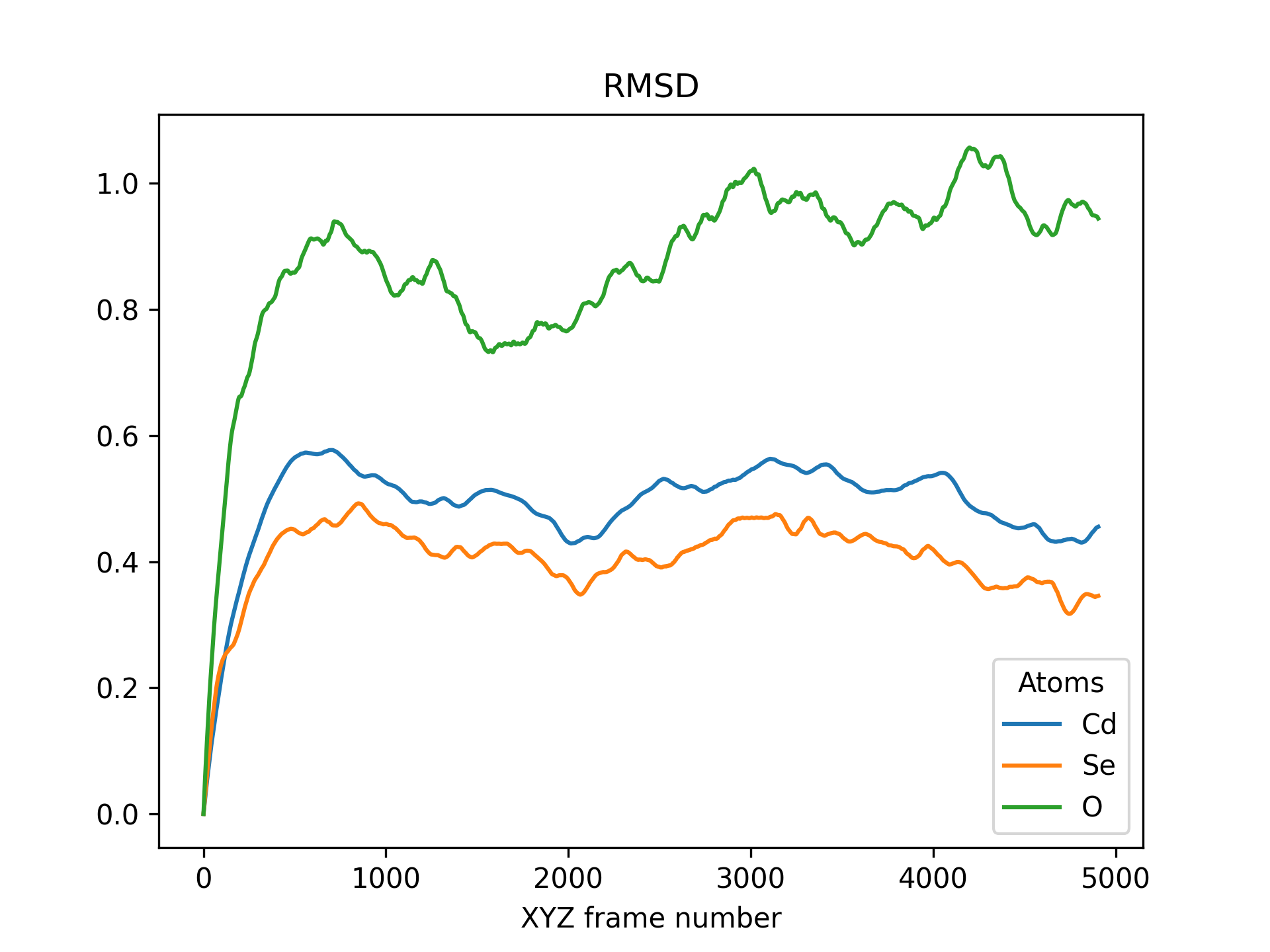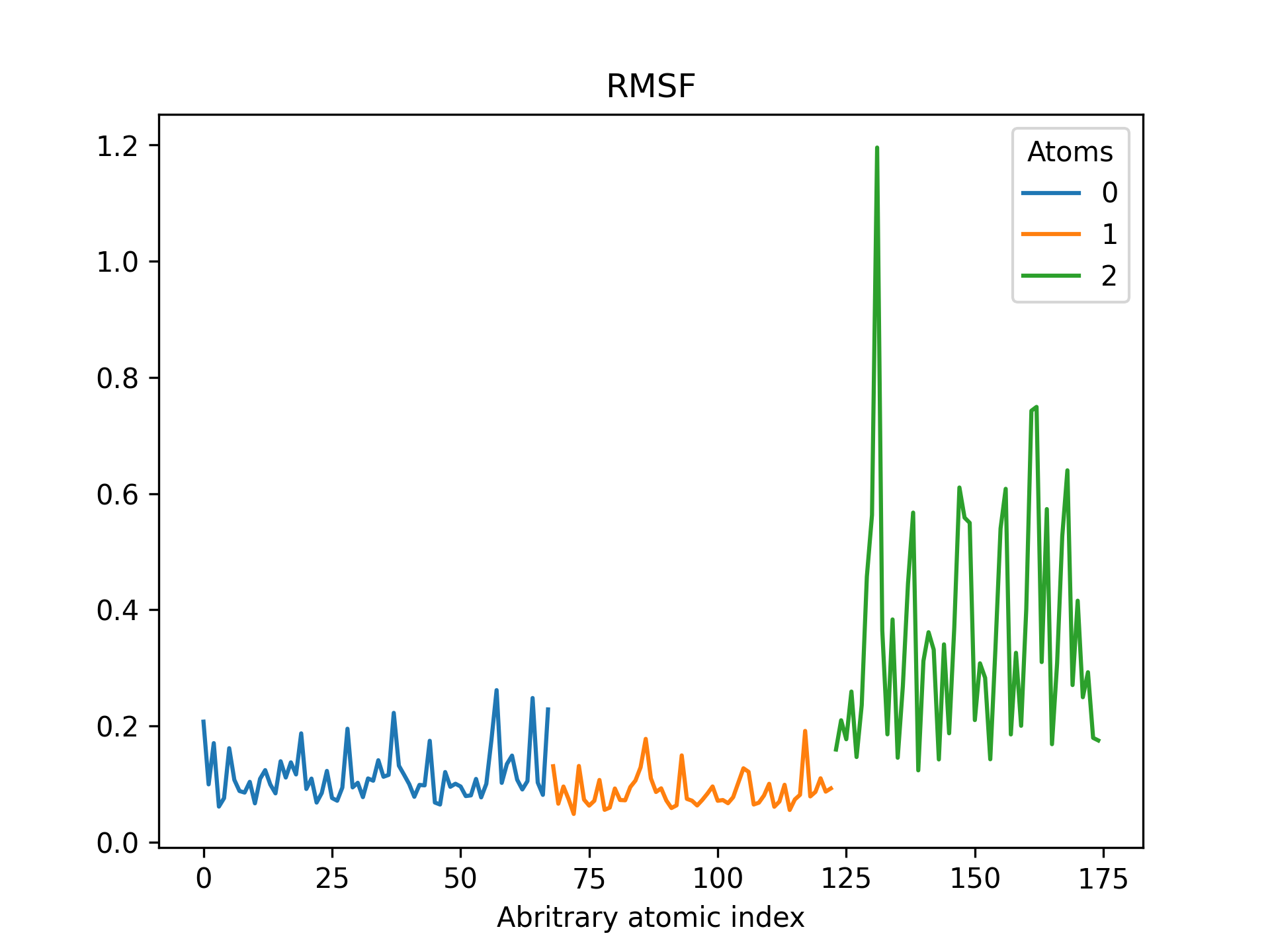# Root Mean Squared Displacement & Fluctuation¶

## Root Mean Squared Displacement¶

The root mean squared displacement (RMSD) represents the average displacement of a set or subset of atoms as a function of time or, equivalently, moleculair indices in a MD trajectory.

$\rho^{\mathrm{RMSD}}(t) = \sqrt{ \frac{1}{N} \sum_{i=1}^{N}\left( \mathbf{r}_{i}(t) - \mathbf{r}_{i}^{\mathrm{ref}}\right )^2 }$

Given a trajectory, mol, stored as a MultiMolecule instance, the RMSD can be calculated with the MultiMolecule.init_rmsd() method using the following command:

>>> rmsd = mol.init_rmsd(atom_subset=None)


The resulting rmsd is a Pandas dataframe, an object which is effectively a hybrid between a dictionary and a NumPy array.

Below is an example RMSD of a CdSe quantum dot pacified with formate ligands. The RMSD is printed for cadmium, selenium and oxygen atoms.

>>> from FOX import MultiMolecule, example_xyz

>>> mol = MultiMolecule.from_xyz(example_xyz)
>>> rmsd = mol.init_rmsd(atom_subset=('Cd', 'Se', 'O'))
>>> rmsd.plot(title='RMSD')## Root Mean Squared Fluctuation¶

The root mean squared fluctuation (RMSD) represents the time-averaged displacement, with respect to the time-averaged position, as a function of atomic indices.

$\rho^{\mathrm{RMSF}}_i = \sqrt{ \left\langle \left(\mathbf{r}_i - \langle \mathbf{r}_i \rangle \right)^2 \right\rangle }$

Given a trajectory, mol, stored as a MultiMolecule instance, the RMSF can be calculated with the MultiMolecule.init_rmsf() method using the following command:

>>> rmsd = mol.init_rmsf(atom_subset=None)


The resulting rmsf is a Pandas dataframe, an object which is effectively a hybrid between a dictionary and a Numpy array.

Below is an example RMSF of a CdSe quantum dot pacified with formate ligands. The RMSF is printed for cadmium, selenium and oxygen atoms.

>>> from FOX import MultiMolecule, example_xyz

>>> mol = MultiMolecule.from_xyz(example_xyz)
>>> rmsd = mol.init_rmsf(atom_subset=('Cd', 'Se', 'O'))
>>> rmsd.plot(title='RMSF')## Discerning shell structures¶

See the MultiMolecule.init_shell_search() method.

>>> from FOX import MultiMolecule, example_xyz
>>> import matplotlib.pyplot as plt

>>> mol = MultiMolecule.from_xyz(example_xyz)
>>> rmsf, rmsf_idx, rdf = mol.init_shell_search(atom_subset=('Cd', 'Se'))

>>> fig, (ax, ax2) = plt.subplots(ncols=2)
>>> rmsf.plot(ax=ax, title='Modified RMSF')
>>> rdf.plot(ax=ax2, title='Modified RDF')
>>> plt.show()The results above can be utilized for discerning shell structures in, e.g., nanocrystals or dissolved solutes, the RDF minima representing transitions between different shells.

• There are clear minima for Se at ~ 2.0, 5.2, 7.0 & 8.5 Angstrom
• There are clear minima for Cd at ~ 4.0, 6.0 & 8.2 Angstrom

With the MultiMolecule.get_at_idx() method it is process the results of MultiMolecule.init_shell_search(), allowing you to create slices of atomic indices based on aforementioned distance ranges.

>>> dist_dict = {}
>>> dist_dict['Se'] = [2.0, 5.2, 7.0, 8.5]
>>> dist_dict['Cd'] = [4.0, 6.0, 8.2]
>>> idx_dict = mol.get_at_idx(rmsf, rmsf_idx, dist_dict)

>>> print(idx_dict)
{'Se_1': ,
'Se_2': [10, 11, 14, 22, 23, 26, 28, 31, 32, 40, 43, 44],
'Se_3': [7, 13, 15, 39, 41, 47],
'Se_4': [1, 3, 4, 6, 8, 9, 12, 16, 17, 19, 21, 24, 30, 33, 35, 37, 38, 42, 45, 46, 48, 50, 51, 53],
'Se_5': [0, 2, 5, 18, 20, 25, 29, 34, 36, 49, 52, 54],
'Cd_1': [25, 26, 30, 46],
'Cd_2': [10, 13, 14, 22, 29, 31, 41, 42, 45, 47, 50, 51],
'Cd_3': [3, 7, 8, 9, 11, 12, 15, 16, 17, 18, 21, 23, 24, 27, 34, 35, 38, 40, 43, 49, 52, 54, 58, 59, 60, 62, 63, 66],
'Cd_4': [0, 1, 2, 4, 5, 6, 19, 20, 28, 32, 33, 36, 37, 39, 44, 48, 53, 55, 56, 57, 61, 64, 65, 67]
}


It is even possible to use this dictionary with atom names & indices for renaming atoms in a MultiMolecule instance:

>>> print(list(mol.atoms))
['Cd', 'Se', 'C', 'H', 'O']

>>> del mol.atoms['Cd']
>>> del mol.atoms['Se']
>>> mol.atoms.update(idx_dict)
>>> print(list(mol.atoms))
['C', 'H', 'O', 'Se_1', 'Se_2', 'Se_3', 'Se_4', 'Se_5', 'Cd_1', 'Cd_2', 'Cd_3']


## The atom_subset argument¶

In the above two examples atom_subset=None was used an optional keyword, one which allows one to customize for which atoms the RMSD & RMSF should be calculated and how the results are distributed over the various columns.

There are a total of four different approaches to the atom_subset argument:

1. atom_subset=None: Examine all atoms and store the results in a single column.

2. atom_subset=int: Examine a single atom, based on its index, and store the results in a single column.

3. atom_subset=str or atom_subset=list(int): Examine multiple atoms, based on their atom type or indices, and store the results in a single column.

4. atom_subset=tuple(str) or atom_subset=tuple(list(int)): Examine multiple atoms, based on their atom types or indices, and store the results in multiple columns. A column is created for each string or nested list in atoms.

It should be noted that lists and/or tuples can be interchanged for any other iterable container (e.g. a Numpy array), as long as the iterables elements can be accessed by their index.

## API¶

MultiMolecule.init_rmsd(mol_subset=None, atom_subset=None, reset_origin=True)[source]

Initialize the RMSD calculation, returning a dataframe.

Parameters: mol_subset (slice) – Perform the calculation on a subset of molecules in this instance, as determined by their moleculair index. Include all $$m$$ molecules in this instance if None. atom_subset (Sequence) – Perform the calculation on a subset of atoms in this instance, as determined by their atomic index or atomic symbol. Include all $$n$$ atoms per molecule in this instance if None. reset_origin (bool) – Reset the origin of each molecule in this instance by means of a partial Procrustes superimposition, translating and rotating the molecules. A dataframe of RMSDs with one column for every string or list of ints in atom_subset. Keys consist of atomic symbols (e.g. "Cd") if atom_subset contains strings, otherwise a more generic ‘series ‘ + str(int) scheme is adopted (e.g. "series 2"). Molecular indices are used as index. pd.DataFrame
MultiMolecule.init_rmsf(mol_subset=None, atom_subset=None, reset_origin=True)[source]

Initialize the RMSF calculation, returning a dataframe.

Parameters: mol_subset (slice) – Perform the calculation on a subset of molecules in this instance, as determined by their moleculair index. Include all $$m$$ molecules in this instance if None. atom_subset (Sequence) – Perform the calculation on a subset of atoms in this instance, as determined by their atomic index or atomic symbol. Include all $$n$$ atoms per molecule in this instance if None. reset_origin (bool) – Reset the origin of each molecule in this instance by means of a partial Procrustes superimposition, translating and rotating the molecules. A dataframe of RMSFs with one column for every string or list of ints in atom_subset. Keys consist of atomic symbols (e.g. "Cd") if atom_subset contains strings, otherwise a more generic ‘series ‘ + str(int) scheme is adopted (e.g. "series 2"). Molecular indices are used as indices. pd.DataFrame
MultiMolecule.init_shell_search(mol_subset=None, atom_subset=None, rdf_cutoff=0.5)[source]

Calculate and return properties which can help determining shell structures.

The following two properties are calculated and returned:

• The mean distance (per atom) with respect to the center of mass (i.e. a modified RMSF).
• A series mapping abritrary atomic indices in the RMSF to the actual atomic indices.
• The radial distribution function (RDF) with respect to the center of mass.
Parameters: mol_subset (slice) – Perform the calculation on a subset of molecules in this instance, as determined by their moleculair index. Include all $$m$$ molecules in this instance if None. atom_subset (Sequence) – Perform the calculation on a subset of atoms in this instance, as determined by their atomic index or atomic symbol. Include all $$n$$ atoms per molecule in this instance if None. rdf_cutoff (float) – Remove all values in the RDF below this value (Angstrom). Usefull for dealing with divergence as the “inter-atomic” distance approaches 0.0 A. Returns the following items: A dataframe holding the mean distance of all atoms with respect the to center of mass. A series mapping the indices from 1. to the actual atomic indices. A dataframe holding the RDF with respect to the center of mass.
static MultiMolecule.get_at_idx(rmsf, idx_series, dist_dict)[source]

Create subsets of atomic indices.

The subset is created (using rmsf and idx_series) based on distance criteria in dist_dict.

For example, dist_dict = {'Cd': [3.0, 6.5]} will create and return a dictionary with three keys: One for all atoms whose RMSF is smaller than 3.0, one where the RMSF is between 3.0 and 6.5, and finally one where the RMSF is larger than 6.5.

Examples

>>> dist_dict = {'Cd': [3.0, 6.5]}
>>> idx_series = pd.Series(np.arange(12))
>>> rmsf = pd.DataFrame({'Cd': np.arange(12, dtype=float)})
>>> get_at_idx(rmsf, idx_series, dist_dict)

{'Cd_1': [0, 1, 2],
'Cd_2': [3, 4, 5],
'Cd_3': [7, 8, 9, 10, 11]
}

Parameters: rmsf (pd.DataFrame) – A dataframe holding the results of an RMSF calculation. idx_series (pd.Series) – A series mapping the indices from rmsf to actual atomic indices. dist_dict (dict [str, list [float]]) – A dictionary with atomic symbols (see rmsf.columns) and a list of interatomic distances. A dictionary with atomic symbols as keys, and matching atomic indices as values. dict [str, list [int]] KeyError – Raised if a key in dist_dict is absent from rmsf.